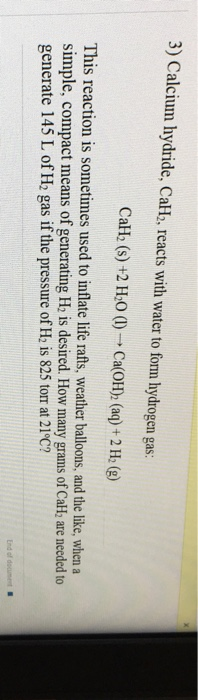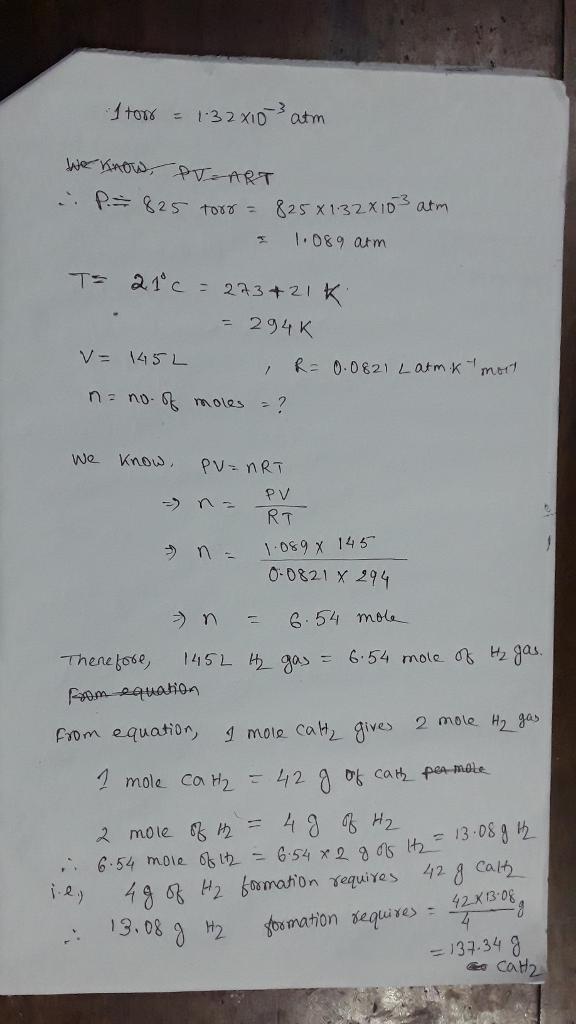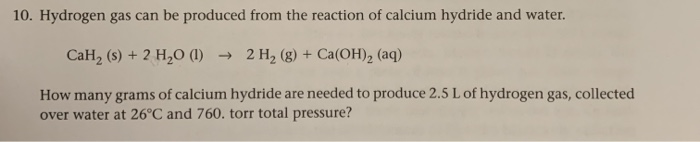# 3) Calcium hydride, Call, reacts with water to fomm lydrogen gas: CaH2 (s) +2 H2O (l)-Ca(OH)2...3) Calcium hydride, Call, reacts with water to fomm lydrogen gas: CaH2 (s) +2 H2O (l)-Ca(OH)2 (aq) + 2 H2 (g) This reaction is sometimes used to inflate life rafts, weather balloons, and the like, when a simple, compact means of generating H is desired. How many gram generate 145 L of H2 gas if the pressure of He is 825 torr at 21°C? s of CalH are needed to#### Earn Coin

Coins can be redeemed for fabulous gifts.

Similar Homework Help Questions
• ### Calcium hydride, CaH2, reacts with water to form hydrogen gas. CaH2(s) + 2 H2O(l) Ca(OH)2(aq) +...

Calcium hydride, CaH2, reacts with water to form hydrogen gas. CaH2(s) + 2 H2O(l) Ca(OH)2(aq) + 2 H2(g) This reaction is sometimes used to inflate life rafts, weather balloons, and the like, where a simple, compact means of generating H2 is desired. How many grams of CaH2 are needed to generate 14.0 L of H2 gas if the pressure of H2 is 730. torr at 27°C? g

• ### Calcium hydride, CaH2, reacts with water to form hydrogen gas: CaH2 (s) + H20 (1) -->...

Calcium hydride, CaH2, reacts with water to form hydrogen gas: CaH2 (s) + H20 (1) --> Ca(OH)2 (aq) + 2 H2 (g) This reaction is sometimes used to inflate life rafts when a simple, compact means of generating H2 is desired. How many grams of CaH2 are needed to generate 115 Lof H2 gas with a pressure of 815 torr at 19 °C? (MMCAH2 = 42.098 g/mol, MMH20 = 18.02 g/mol, MMCA(OH)2 = 74.098 g/mol, MMH2 = 2.02 g/mol)

• ### Please any help would be greatly appreciated.

Calcium hydride, CaH2, reacts with water to form hydrogen gas.CaH2(s) + 2 H2O(l) Ca(OH)2(aq) + 2 H2(g)This reaction is sometimes used to inflate life rafts, weather balloons, and the like, where a simple, compact means of generating H2 is desired. How many grams ofCaH2 are needed to generate 15.0 L of H2 gas if the pressure of H2 is 740. torr at 16°C?

• ### 6. a. Calculate the density of sulfur hexafluoride gas at 709 torr and 23 C. b....

6. a. Calculate the density of sulfur hexafluoride gas at 709 torr and 23 C. b. Calculate the molar mass of a vapor that has a density of 7.125 g/L at 11 ∘C and 745 torr . c. Calcium hydride, CaH2, reacts with water to form hydrogen gas: CaH2(s)+2H2O(l)→Ca(OH)2(aq)+2H2(g) This reaction is sometimes used to inflate life rafts, weather balloons, and the like, where a simple, compact means of generating H2 is desired. How many grams of CaH2 are needed...

• ### Gas pressure

Calcium hydride, CaH2, reacts with water to form hydrogen gas. CaH2(s) + 2 H2O(l) Ca(OH)2(aq) + 2 H2(g) This reaction is sometimes used to inflate life rafts, weatherballoons, and the like, where a simple, compact means of generating H2 is desired. How many grams of CaH2 are needed to generate 16.0 L of H2 gas if the pressure of H2is 750. torr at 25°C? ___ g

• ### Calcium hydride (CaH2) reacts with water to form hydrogen gas: CaH2(s) + 2H2O(l) → Ca(OH)2(aq) +...

Calcium hydride (CaH2) reacts with water to form hydrogen gas: CaH2(s) + 2H2O(l) → Ca(OH)2(aq) + 2H2(g) How many grams of CaH2 are needed to generate 45.0 L of H2 gas at a pressure of 0.888 atm and a temperature of 32 °C?

• ### Calcium hydride (CaH2) reacts with water to form hydrogen gas: CaH2 (s)+2H2O(i)-->Ca(OH)2(aq) +2H2 (g). what volume...

Calcium hydride (CaH2) reacts with water to form hydrogen gas: CaH2 (s)+2H2O(i)-->Ca(OH)2(aq) +2H2 (g). what volume of H2 gas at 40*C and 750 torr could be produced from 0.710kg of CaH2?

• ### (6 points) Calcium hydride reacts with water as follows: CaH2(s) + H2O(l) → Ca(OH)2(aq) + H2(g)...

(6 points) Calcium hydride reacts with water as follows: CaH2(s) + H2O(l) → Ca(OH)2(aq) + H2(g) How many grams of CaH2 are needed to produce 4.1 L H2(g) at 0.97 atm and 12 °C?

• ### #CaH_2(s) + 2 H_2O(l)= Ca(OH)_2(aq) + 2 H_2(g)# How many grams of CaH2 are needed to generate 10.0 L of H2 gas if the pressure of H2 is 720. torr at 16°C?

This reaction is sometimes used to inflate life rafts, weather balloons, and the like, where a simple, compact means of generating H2 is desired.

• ### 10. Hydrogen gas can be produced from the reaction of calcium hydride and water. CaH, (s)...10. Hydrogen gas can be produced from the reaction of calcium hydride and water. CaH, (s) + 2 H20 (1) ► 2 H2(g) + Ca(OH)2 (aq) How many grams of calcium hydride are needed to produce 2.5 L of hydrogen gas, collected over water at 26°C and 760. torr total pressure?

Free Homework App